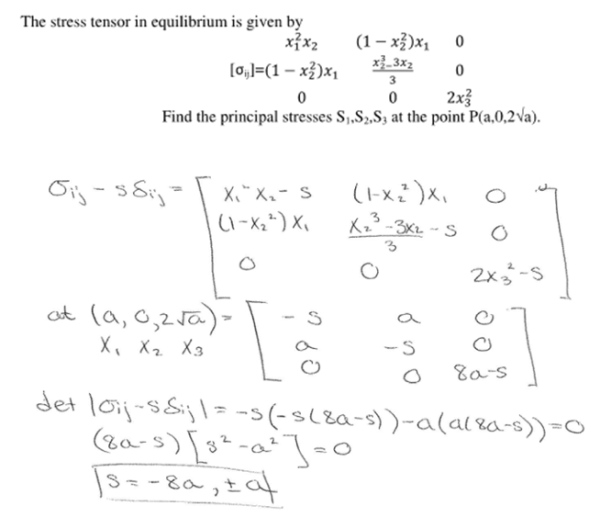The stress tensor in equilibrium is given by the following matirx. Find the principal stresses S1, S2, S3 at the pint P(a,0,2a^1/2).The stress tensor in equilibrium is given by the following matirx. Find the principal stresses S1, S2, S3 at the pint P(a,0,2a^1/2).

biomechanics page 1 biomechanics biomechanics biomechanics biomechanics biomechanics biomechanics biomechanics biomechanics page 2